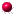# Gamma-ray Burst 150725A(All information courtesy of the instrument teams.)Previous IAU Circulars

• noneResults of Observations
• GCN Circular #18062
T. Masumitsu, H. Negoro, (Nihon U.), T. Sakamoto (AGU), S. Ueno, H. Tomida, S. Nakahira, M. Kimura, M. Ishikawa, Y. E. Nakagawa (JAXA), T. Mihara, M. Sugizaki, M. Serino, M. Shidatsu, J. Sugimoto, T. Takagi, M. Matsuoka (RIKEN), N. Kawai, T. Yoshii, Y. Tachibana (Tokyo Tech), A. Yoshida, T. Sakamoto, Y. Kawakubo, H. Ohtsuki (AGU), H. Tsunemi, R.Imatani (Osaka U.), M. Nakajima, K. Tanaka (Nihon U.), Y. Ueda, T. Kawamuro, T. Hori (Kyoto U.), Y. Tsuboi, S. Kanetou (Chuo U.), M. Yamauchi, D. Itoh (Miyazaki U.), K. Yamaoka (Nagoya U.), M. Morii (ISM) report on behalf of the MAXI team:
The MAXI/GSC nova alert system triggered a bright uncatalogued X-ray transient source at UT 08:45:00 UT. Assuming that the source flux was constant over the transit, we obtain the source position at
(R.A., Dec) = (220.422 deg, -2.421 deg) = (14 41 41, -02 25 15) (J2000)
with a statistical 90% C.L. elliptical error region with long and short radii of 0.11 deg and 0.09 deg, respectively. The roll angle of long axis from the north direction is 117.0 deg counterclockwise. There is an additional systematic uncertainty of 0.1 deg (90% containment radius). The X-ray flux averaged over the scan was 1491 +- 107 mCrab (4-10keV, 1 sigma error).
Without assumptions on the source constancy, we obtain a rectangular error box for the transient source with the following corners:
```(R.A., Dec) = (220.229 deg, -2.781 deg) = (14 40 55, -02 46 51) (J2000)
(R.A., Dec) = (220.058 deg, -2.639 deg) = (14 40 14, -02 38 20) (J2000)
(R.A., Dec) = (220.615 deg, -2.060 deg) = (14 42 27, -02 03 36) (J2000)
(R.A., Dec) = (220.786 deg, -2.201 deg) = (14 43 08, -02 12 04) (J2000)
```
There was no significant excess flux in the previous transit at 07:12 UT with an upper limit of 20 mCrab.
• GCN Circular #18073
S. Golenetskii, R.Aptekar, D. Frederiks, V. Pal'shin, P. Oleynik, M. Ulanov, D. Svinkin, A. Tsvetkova, A.Lysenko, and T. Cline on behalf of the Konus-Wind team, report:
The long-duration GRB 150725A (Masumitsu et al., GCN Circ. 18062) triggered Konus-Wind at T0=31454.410 s UT (08:44:14.410), ~15 s before the MAXI trigger.
The KW light curve measured in the triggered mode shows multiple bright pulses in the interval from ~T0-0.5 s to ~T0+60 s. The emission is visible up to ~10 MeV.
As observed by Konus-Wind, the burst had a fluence of 1.55(-0.10,+0.09)x10^-4 erg/cm2, and a 64-ms peak flux, measured from T0+51.456 s, of 1.35(-0.23,+0.17)x10^-5 erg/cm2/s (both in the 20 keV - 10 MeV energy range).
The time-averaged spectrum (measured from T0 to T0+58.112 s) is best fit in the 20 keV - 15 MeV range by the GRB (Band) function with the following model parameters: the low-energy photon index alpha = -0.84 (-0.07,+0.08), the high energy photon index beta = -2.32 (-0.13,+0.10), the peak energy Ep = 326 (-24,+28) keV, chi2 = 125/97 dof.
The spectrum near the peak count rate (measured from T0+51.200 to T0+58.112 s) is best fit in the 20 keV - 15 MeV range by the GRB (Band) function with the following model parameters: the low-energy photon index alpha = -0.77 (-0.23,+0.26), the high energy photon index beta = -2.29 (-0.52,+0.18), the peak energy Ep = 270 (-53,+86) keV, chi2 = 92/97 dof.
The Konus-Wind light curve of this GRB is available at http://www.ioffe.ru/LEA/GRBs/GRB150725_T31454/
All the quoted errors are at the 90% confidence level. All the quoted values are preliminary.

Back to JG's homepage
Jochen Greiner, last update: 29-Jul-2015 [Disclaimer]